# How To: Extract Table From Image In Python (OpenCV & OCR)

In this guide, we will use OpenCV and TesseractOCR to extract a table from an image in Python. We will use an image of a nutrition label from the back of a box of chocolates. We will assume that you are making a project where these types of nutrition tables need to be digitized.

Note: If you try to use this code as-is for your situation, you might find that it does not work well. That is because there are a lot of small things you can tweak and perfect as per your situation. After you understand the code, you will be in a very good place to do this. At the end of the guide, I have also suggested some further improvements you can take.

## A Video Of The Final Product & A Visual Overview Of The Process

Have a look at this video, it should help you to see the entire process in action.

## The Code

The code comprises 3 classes for the 3 stages of the process. I have created a public repo on GitHub and uploaded the code there.

## Stage A: Detecting the table

Stage A is all about looking at the image and finding the area that is most likely the table. This is done by simply looking for the biggest “box” in the image.

Let’s look at the inputs and outputs of Stage A before we proceed:Above is the original image.

The output after Stage A is as follows: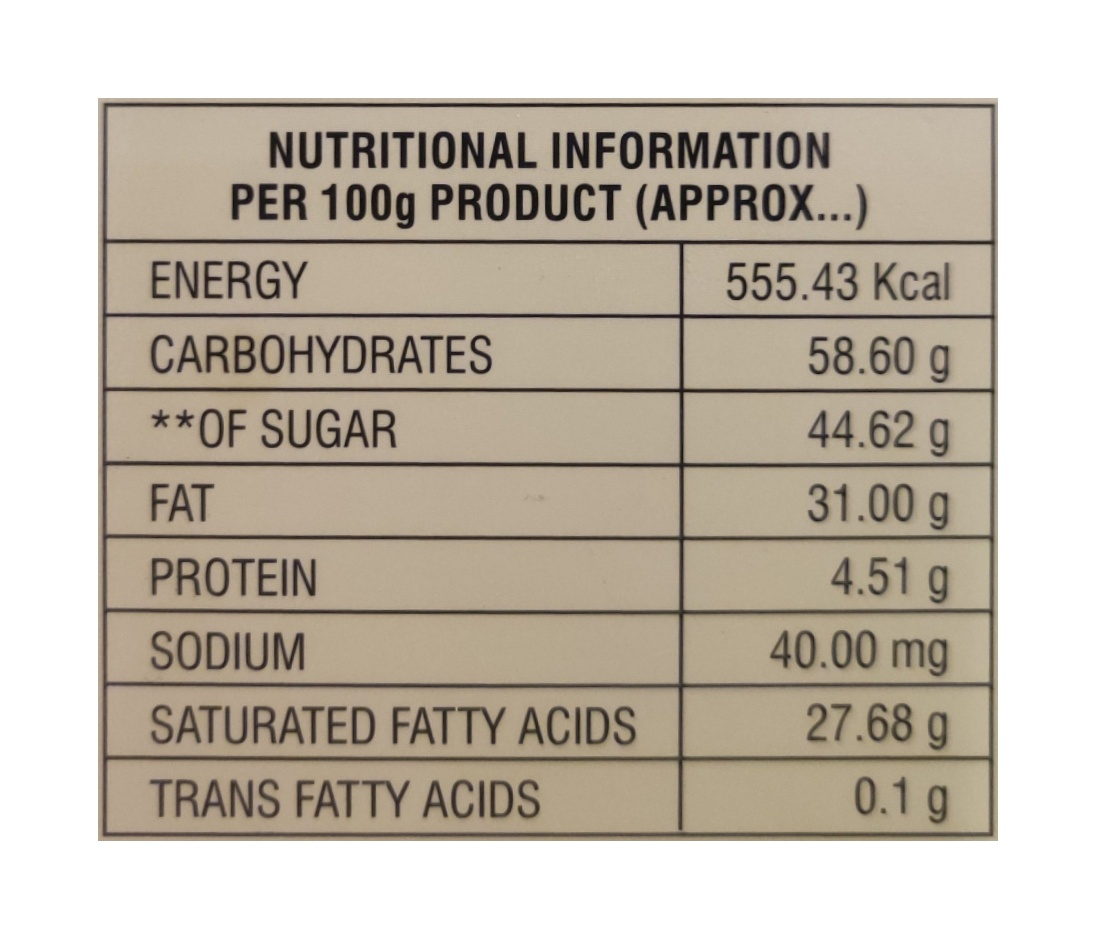So, let’s start looking at how we can achieve the above.

### Stage A: Overall Plan Simplified

Basically, we are going to take the image and thicken all the lines. Once we do that, we find all the “contours“. Below is how the official OpenCV docs explain “contours”:

What are contours?

Contours can be explained simply as a curve joining all the continuous points (along the boundary), having same color or intensity. The contours are a useful tool for shape analysis and object detection and recognition.

Once, we have all the contours, we will find the largest rectangular one and hope that that’s our table.

The reason we are “thickening” our lines first, is that we are trying to make sure we get a strong and clear contour for the outside of our table. If we do not do this, the internal cell boxes might get confused with the outer box. (You can try and eliminate this thickening step in order to see if it works for your use case.)

Finally, once we have the largest rectangle, we are going to correct the perspective using OpenCVs built-in functions.

### Step A1: Preprocessing

This step is composed of 4 sub-steps. Below I am going to list out the steps, why I am doing them, and also links to the official docs so that you can go in deeper into the subjects if you need.

Step No Name Why We Are Doing This? Link To Official Docs
1 Grey-scaling We don’t care about the color info. Getting rid of it makes processing faster. Link
2 Thresholding We don’t even care about the shades of grey. So, we are reducing the image to just “black” or “white” pixels. Also makes things faster and is needed for further operations. Link
3 Inverting The image is such that the text is black and the background is white. We need to invert this so that we can apply the next operation. Link
4 Dilating Here we are going to make all the lines and any shapes in the image thicker. This will help us to correctly identify the “contours” and hopefully the “contour” that makes up the largest box. We are hoping that the largest box is the table. Link

Below is how the image changes as it goes through the above steps:

Below is the Python code for doing the above transformations. The names of the functions should make it clear what is going on. In order to understand some of the parameters etc, you will need to look at the official docs links provided in the above table:

```def convert_image_to_grayscale(self):
self.grayscale_image = cv2.cvtColor(self.image, cv2.COLOR_BGR2GRAY)

def threshold_image(self):
self.thresholded_image = cv2.threshold(self.grayscale_image, 0, 255, cv2.THRESH_BINARY + cv2.THRESH_OTSU)

def invert_image(self):
self.inverted_image = cv2.bitwise_not(self.thresholded_image)

def dilate_image(self):
self.dilated_image = cv2.dilate(self.inverted_image, None, iterations=5)```

### Step A2: Find & Filter The Contours To Find The Largest Rectangular Contour

Now we are going to do the following:

1. Ask OpenCV to find all the contours.
2. Loop through the contours and leave only “rectangular” contours.
3. Find the largest contour by area (this one hopefully has our table)

When we do the above steps, this is what it looks like visually:

Note That: Even tho we are doing the work of finding the contours etc on the pre-processed image we have generated above, the printing of the contours is done on the original image. This is done because we cannot draw “Green” lines on a binary black-and-white image. There is no way to represent green in black and white.

Below is what the code of this section looks like:

```def find_contours(self):
self.contours, self.hierarchy = cv2.findContours(self.dilated_image, cv2.RETR_TREE, cv2.CHAIN_APPROX_SIMPLE)
# Below lines are added to show all contours
# This is not needed, but it is useful for debugging
self.image_with_all_contours = self.image.copy()
cv2.drawContours(self.image_with_all_contours, self.contours, -1, (0, 255, 0), 3)

def filter_contours_and_leave_only_rectangles(self):
self.rectangular_contours = []
for contour in self.contours:
peri = cv2.arcLength(contour, True)
approx = cv2.approxPolyDP(contour, 0.02 * peri, True)
if len(approx) == 4:
self.rectangular_contours.append(approx)
# Below lines are added to show all rectangular contours
# This is not needed, but it is useful for debugging
self.image_with_only_rectangular_contours = self.image.copy()
cv2.drawContours(self.image_with_only_rectangular_contours, self.rectangular_contours, -1, (0, 255, 0), 3)

def find_largest_contour_by_area(self):
max_area = 0
self.contour_with_max_area = None
for contour in self.rectangular_contours:
area = cv2.contourArea(contour)
if area > max_area:
max_area = area
self.contour_with_max_area = contour
# Below lines are added to show the contour with max area
# This is not needed, but it is useful for debugging
self.image_with_contour_with_max_area = self.image.copy()
cv2.drawContours(self.image_with_contour_with_max_area, [self.contour_with_max_area], -1, (0, 255, 0), 3)```

Note that in all the functions above, the last few lines are about showing the contours of the image. This is not strictly needed. But, it’s given anyways so that you can debug if something is not working as you expect.

As you can see above in the first function, we are doing all the work of finding the contours on the “dilated” image. And when showing the contours we are drawing them on a copy of the original image.

Below are some links to the official docs that you might need to understand the above code:

### Step A3: Perspective Correction

As you can see above, we have the largest rectangle. Now we need to isolate only that part of the image and also need to fix the perspective. In order to do this, we will need to give OpenCV all the info about what the coordinates of the points on the old image are and how they need to map to the new transformed image. In order to do this, we will need to do the following:

1. Order the 4 points in the contour of the largest rectangle we found above.
2. Based on the points, and width and height of the rectangle, estimate the size of the new image to be generated
3. Finally, apply the perspective transform

Most of this work is just code and logic. So there is not much to show in terms of images. But below I have drawn the 4 points that make up the corners of the rectangle on the original image. The code below shows how to do this. This will help with debugging.Let’s look at the code. The naming of the variables and methods should make it fairly clear what’s going on:

```def order_points_in_the_contour_with_max_area(self):
self.contour_with_max_area_ordered = self.order_points(self.contour_with_max_area)
# The code below is to plot the points on the image
# it is not required for the perspective transform
self.image_with_points_plotted = self.image.copy()
for point in self.contour_with_max_area_ordered:
point_coordinates = (int(point), int(point))
self.image_with_points_plotted = cv2.circle(self.image_with_points_plotted, point_coordinates, 10, (0, 0, 255), -1)

def calculate_new_width_and_height_of_image(self):
existing_image_width = self.image.shape
existing_image_width_reduced_by_10_percent = int(existing_image_width * 0.9)

distance_between_top_left_and_top_right = self.calculateDistanceBetween2Points(self.contour_with_max_area_ordered, self.contour_with_max_area_ordered)
distance_between_top_left_and_bottom_left = self.calculateDistanceBetween2Points(self.contour_with_max_area_ordered, self.contour_with_max_area_ordered)

aspect_ratio = distance_between_top_left_and_bottom_left / distance_between_top_left_and_top_right

self.new_image_width = existing_image_width_reduced_by_10_percent
self.new_image_height = int(self.new_image_width * aspect_ratio)

def apply_perspective_transform(self):
pts1 = np.float32(self.contour_with_max_area_ordered)
pts2 = np.float32([[0, 0], [self.new_image_width, 0], [self.new_image_width, self.new_image_height], [0, self.new_image_height]])
matrix = cv2.getPerspectiveTransform(pts1, pts2)
self.perspective_corrected_image = cv2.warpPerspective(self.image, matrix, (self.new_image_width, self.new_image_height))

# Below are helper functions
def calculateDistanceBetween2Points(self, p1, p2):
dis = ((p2 - p1) ** 2 + (p2 - p1) ** 2) ** 0.5
return dis

def order_points(self, pts):
# initialzie a list of coordinates that will be ordered
# such that the first entry in the list is the top-left,
# the second entry is the top-right, the third is the
# bottom-right, and the fourth is the bottom-left
pts = pts.reshape(4, 2)
rect = np.zeros((4, 2), dtype="float32")

# the top-left point will have the smallest sum, whereas
# the bottom-right point will have the largest sum
s = pts.sum(axis=1)
rect = pts[np.argmin(s)]
rect = pts[np.argmax(s)]

# now, compute the difference between the points, the
# top-right point will have the smallest difference,
# whereas the bottom-left will have the largest difference
diff = np.diff(pts, axis=1)
rect = pts[np.argmin(diff)]
rect = pts[np.argmax(diff)]

# return the ordered coordinates
return rect```

After the perspective transformation, we have an image that looks like this: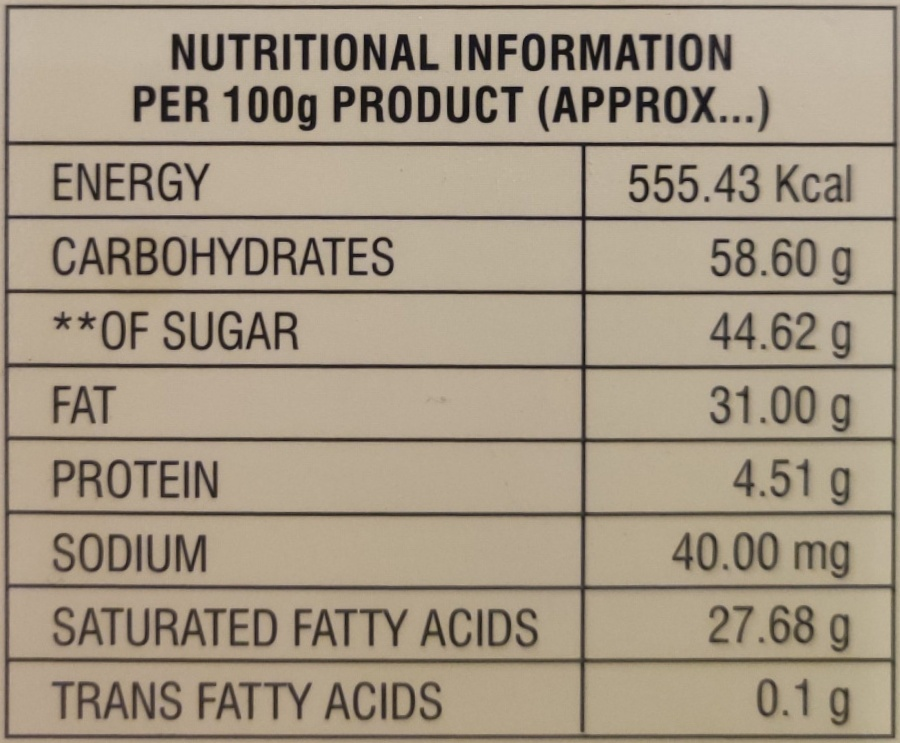One last thing we will do is add some padding to the image. This will be needed in the next stage when we remove the lines. Without this, I have noticed that the lines do not get removed fully.

```def add_10_percent_padding(self):
image_height = self.image.shape

Here are the official docs for the method used: Adding borders to your images

When we are done with this, we get an image that looks like this:## Stage B: Removing The Lines

This stage is all about getting rid of the lines of the table. This will help us to have a clear image for the OCR process. The only things left of the image, in the end, will be the text in the table cells.

Let’s look at how the end result will look at the end of this stage: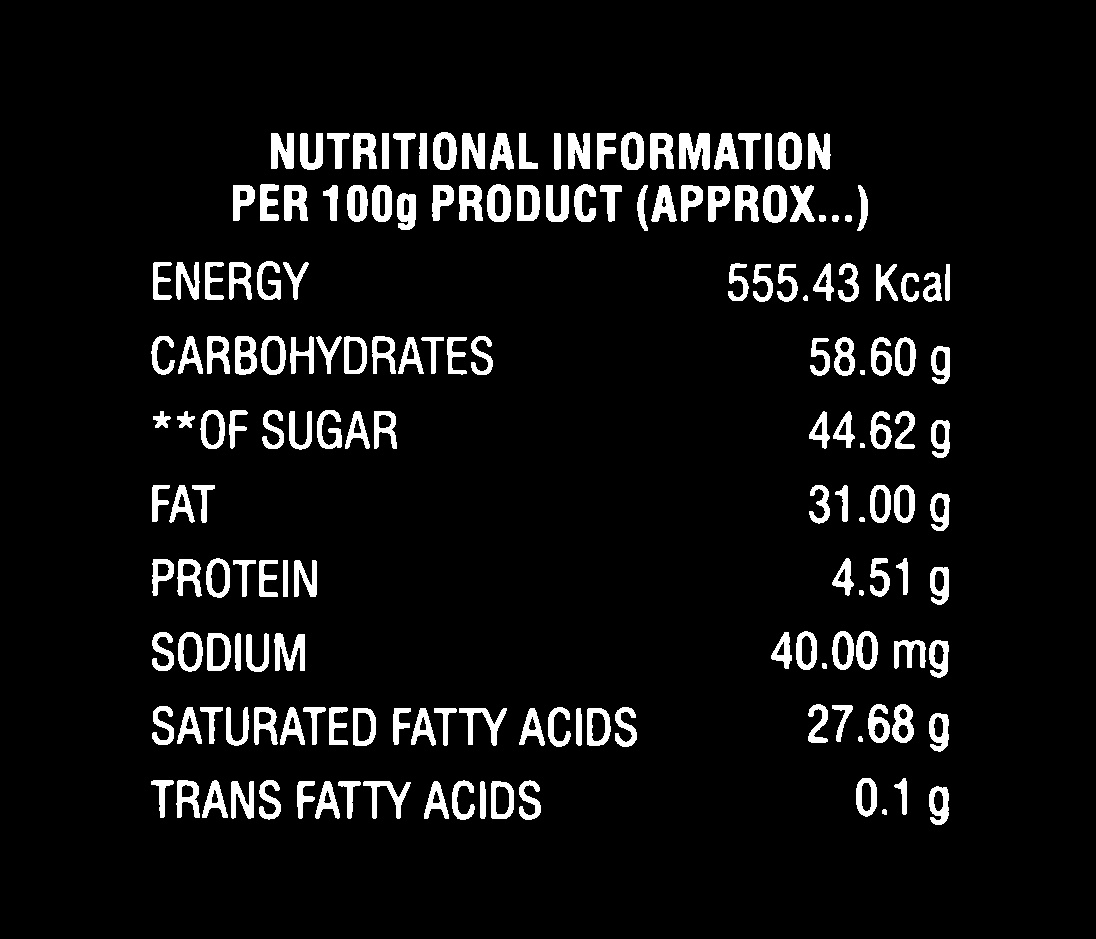### Step B1: Preprocessing

This is very similar to the pre-processing we did in the last stage.

Note: You can even choose to skip this and use an image that was generated from the last stage. The reason why I have done this again is for clarity and also so that the Python class that makes up this stage can be used modularly given an image that only contains a table and nothing else.

This stage takes a full-color image from the last stage along with the padding and converts it into an inverted binary image.

Step No Name Why We Are Doing This? Link To Official Docs
1 Grey-scaling We don’t care about the color info. Getting rid of it makes processing faster. Link
2 Thresholding We don’t even care about the shades of grey. So, we are reducing the image to just “black” or “white” pixels. Also makes things faster and is needed for further operations. Link
3 Inverting The image is such that the text is black and the background is white. We need to invert this so that we can apply the next operation. Link

Below is how the image changes as it goes through these stages:

Below is the code:

```def grayscale_image(self):
self.grey = cv2.cvtColor(self.image, cv2.COLOR_BGR2GRAY)

def threshold_image(self):
self.thresholded_image = cv2.threshold(self.grey, 127, 255, cv2.THRESH_BINARY)

def invert_image(self):
self.inverted_image = cv2.bitwise_not(self.thresholded_image)```

### Step B2: Eroding Vertical Lines

In order to understand how the verticle lines and all the text is eroded away, you will have to properly understand the concept of “erosion” and “dilation”.

I have gathered some of the best videos from YouTube on this topic below. After watching these, you will have a better sense of what is going on:

Now, having watched the above, it should be fairly clear what a “kernel” is in the context of erosion and dilation. Basically, it’s a shape that is taken over the images and used to transform the underlying image by removing or adding pixels to the original image.

Now, with that, the following code for removing all the verticle lines should make some sense:

```def erode_vertical_lines(self):
hor = np.array([[1,1,1,1,1,1]])
self.vertical_lines_eroded_image = cv2.erode(self.inverted_image, hor, iterations=10)
self.vertical_lines_eroded_image = cv2.dilate(self.vertical_lines_eroded_image, hor, iterations=10)```

As you can see above, we are creating a “kernel” that is just a horizontal line. Then we are eroding everything that matches the kernel. Once that is done, we are dilating what is left. We are doing this second step because the lines of the table become a little too short after the erosion step. So, we are bringing them back.

At the end of this process, the image looks something like this: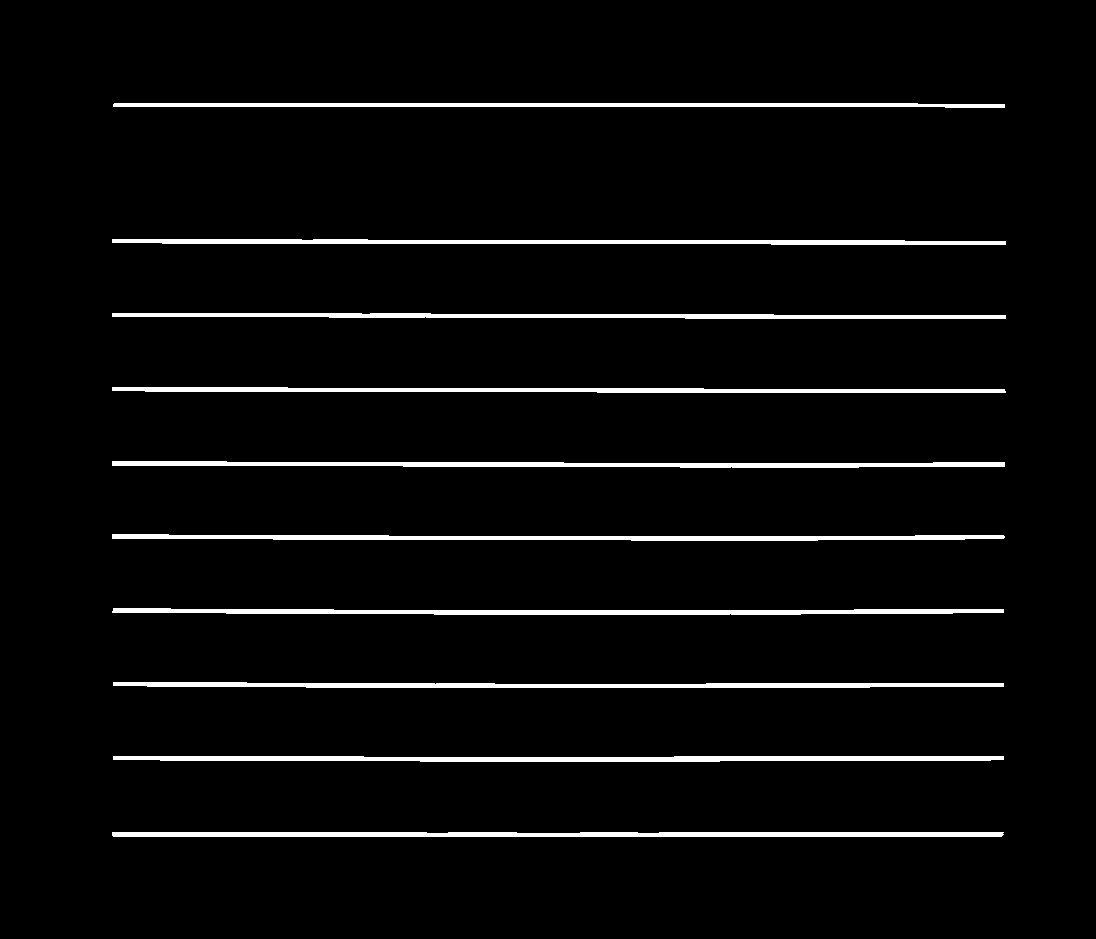This process might seem a little too magical. So, I suggest that you play with the different lines of the code and try out different things like:

1. What happens when you put in a different kernel
2. What happens if you do not dilate after you erode

Here are the official OpenCV docs about erosion and dilation.

They also have a nice tutorial on the subject called: Extract horizontal and vertical lines by using morphological operations

### Step B3: Eroding Horizontal Lines

Next, we are going to use a similar process to erode away the horizontal lines. The explanation is the same as the above so I will not go over it again.

Below is the code:

```def erode_horizontal_lines(self):
ver = np.array([,
,
,
,
,
,
])
self.horizontal_lines_eroded_image = cv2.erode(self.inverted_image, ver, iterations=10)
self.horizontal_lines_eroded_image = cv2.dilate(self.horizontal_lines_eroded_image, ver, iterations=10)```

After the above code runs, we are left with the following image:### Step B4: Combining Vertical And Horizontal Lines

Now, we will combine the horizontal and vertical lines using a simple “add” operation. It just adds the white pixels in both images:

```def combine_eroded_images(self):

The result of the above is: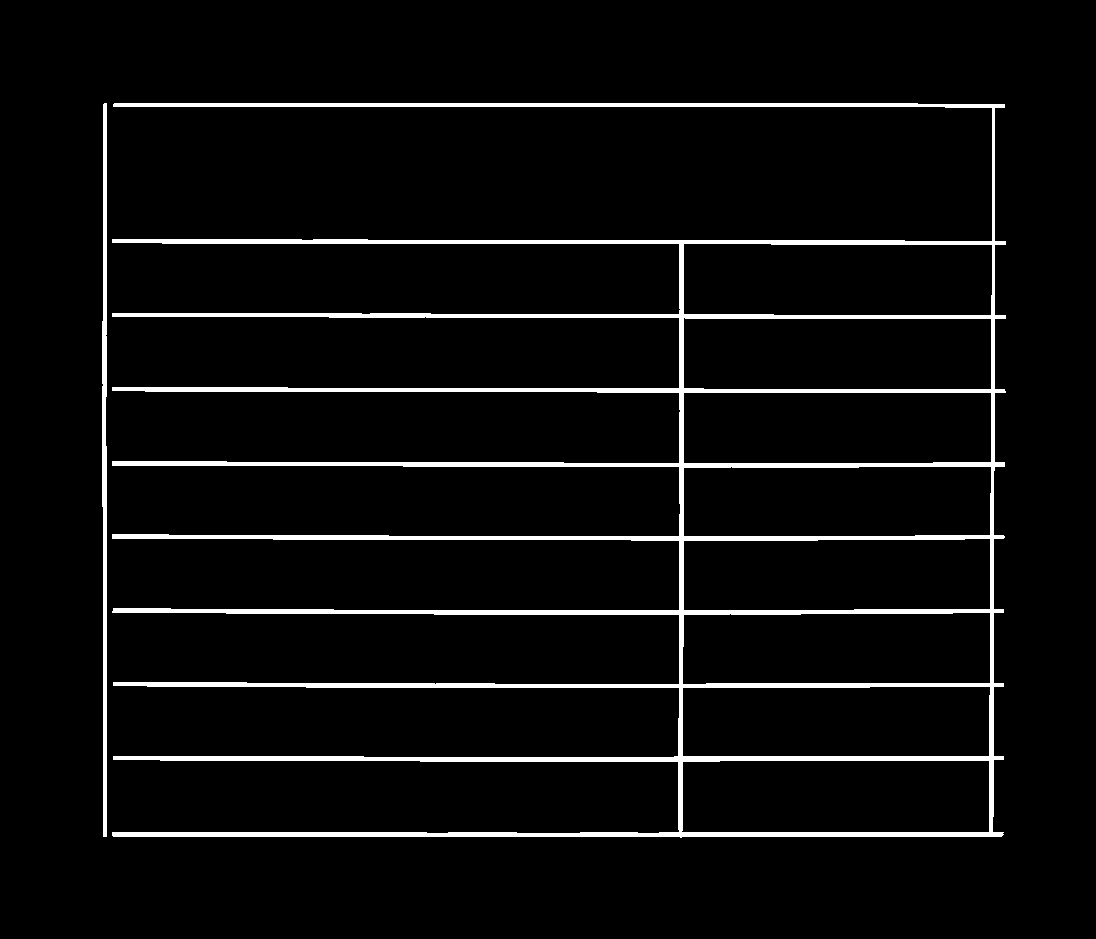Next, we are going to use “dilate” once again to “thicken” these lines.

Why are we thickening? Because just like we did “add” above, we can also do “subtract” and get rid of any areas covered with “white”. But, before we do that, we are making the lines nice and thick so that they easily cover the underlying lines.

So, in order to thicken things up, we use the following code:

```def dilate_combined_image_to_make_lines_thicker(self):
kernel = cv2.getStructuringElement(cv2.MORPH_RECT, (2, 2))
self.combined_image_dilated = cv2.dilate(self.combined_image, kernel, iterations=5)```

Here you can see we are using getStructuringElement in order to create a nice simple rectangular kernel. The simple kernel will go over the image and thicken things up. Something like the video you might have seen above.

At the end of this, we get an image that looks like this:### Step B5: Removing The Lines

This part is easy. Now that we have an image that is only made up of the lines of the table, we can do a “subtract” and get an image without the lines.

The code for this is:

```def subtract_combined_and_dilated_image_from_original_image(self):
self.image_without_lines = cv2.subtract(self.inverted_image, self.combined_image_dilated)```

With this, we get an image that looks like this: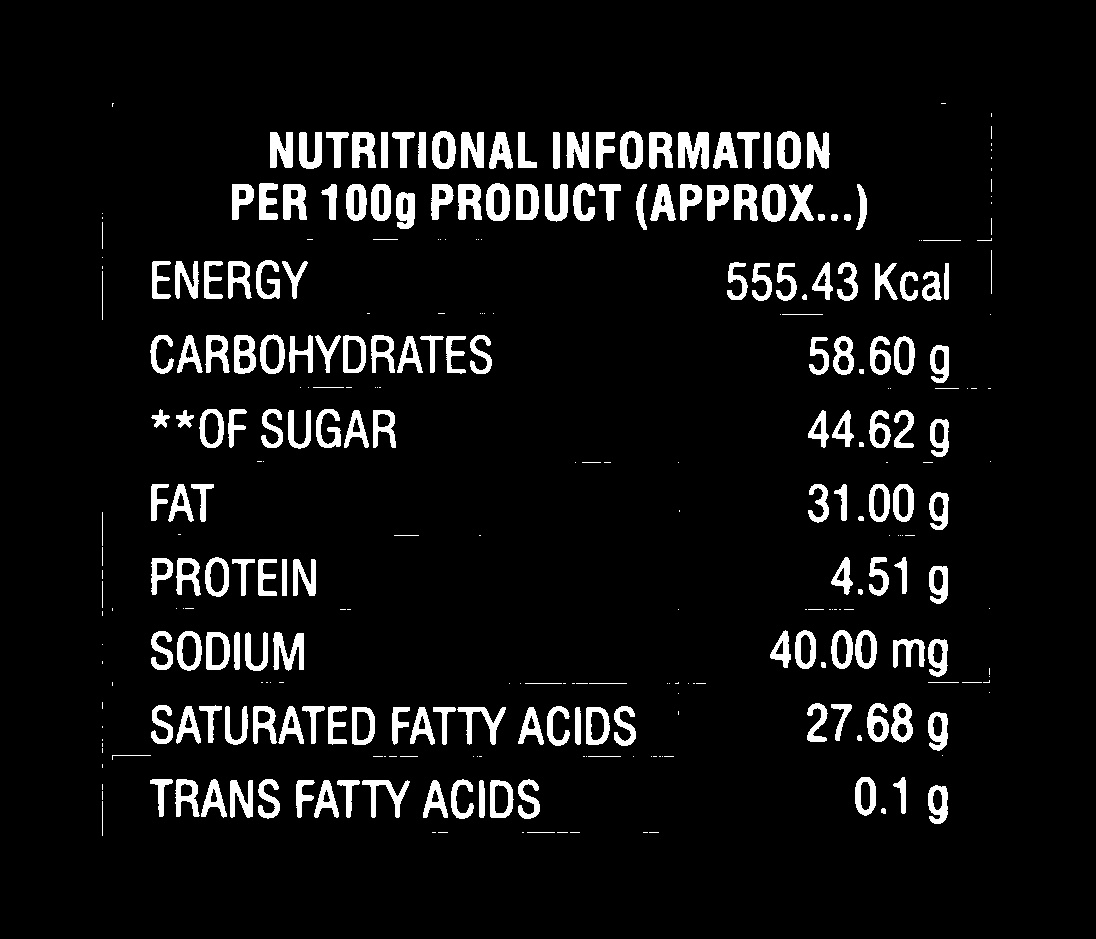I have put a bigger-than-usual image above so that you can notice the problem. The lines are gone (sort of) but there are these little line fragments here and there. We can get rid of those too with a little bit of noise removal:

```def remove_noise_with_erode_and_dilate(self):
kernel = cv2.getStructuringElement(cv2.MORPH_RECT, (2, 2))
self.image_without_lines_noise_removed = cv2.erode(self.image_without_lines, kernel, iterations=1)
self.image_without_lines_noise_removed = cv2.dilate(self.image_without_lines_noise_removed, kernel, iterations=1)```

Here once again we will use erode and dilate. And we are using the same simple kernel we used above. The net effect is that all the little 1, 2 pixel thick bits get completely eroded away. The thicker areas stay. And once they are eroded away, we can dilate and thicken the text and other areas that the erosion ate away.

The final effect is an image that is clean. All the little noise is gone. Only the text remains. We are done with this stage and are ready for the final OCR stage:## Stage C: Finding the cells & extracting the text using OCR

### Stage C: High-Level Plan

Below is the high-level plan:

1. Now that we have an image with only text, we are going to convert all the text into blobs. (Using dilation as usual).
2. Then we are going to use findContours (which we have seen above) to find where all the blobs are.
3. Then we are going to draw bounding boxes around the blobs.
4. Then we will split up the image into little boxes of just the words.
5. We will send each of these image slices to the OCR tool (we will use free Tesseract OCR) in this case. We will get back the text version of the word.
6. Finally, we use a little logic to figure out the rows and columns of the table and we construct the whole thing in terms of a CSV

Let’s look at how to execute the above plan.

### Step C1: Use Dilation To Convert The Words Into Blobs

By this point, (since we have seen a lot of dilation already), what is going on in this step should be clear:

First, let’s look at the code:

```def dilate_image(self):
kernel_to_remove_gaps_between_words = np.array([
[1,1,1,1,1,1,1,1,1,1],
[1,1,1,1,1,1,1,1,1,1]
])
self.dilated_image = cv2.dilate(self.thresholded_image, kernel_to_remove_gaps_between_words, iterations=5)
simple_kernel = np.ones((5,5), np.uint8)
self.dilated_image = cv2.dilate(self.dilated_image, simple_kernel, iterations=2)```

As you can see we have used a long horizontal kernel. That helps us dilate the words and turn them into horizontal smudges. (Look at the image below).

Then we also used a square kernel just to fill in any gaps.

The end result of this is something like this:### Step C2: Find The Contours Of The Blobs

Next, we need to find all these smudges using the findContours method. This is also something we have done before in the first stage.

Below is the code to find the contours and draw them on the original image for the purposes of visualization.

```def find_contours(self):
result = cv2.findContours(self.dilated_image, cv2.RETR_TREE, cv2.CHAIN_APPROX_SIMPLE)
self.contours = result
# The code below is for visualization purposes only.
# It is not necessary for the OCR to work.
self.image_with_contours_drawn = self.original_image.copy()
cv2.drawContours(self.image_with_contours_drawn, self.contours, -1, (0, 255, 0), 3)```

After this, we will have an image that looks like this: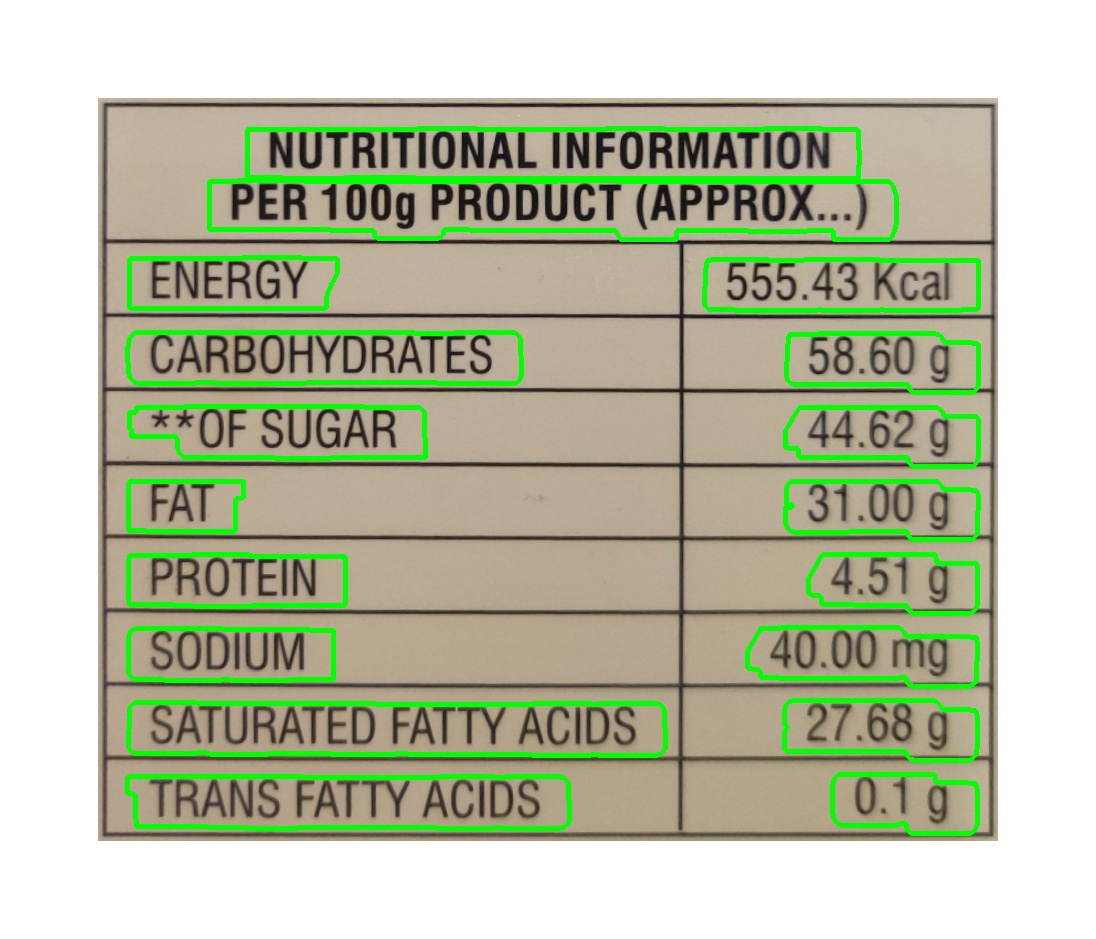### Step C3: Convert The Blobs Into Bounding Boxes

Next, we are going to use a new OpenCV function called: boundingRect (Search for the function on this page of the official docs)

The function takes in the contour (which is made up of many points) and reduces it to a box that can fully enclose the contour shape.

Below is the code:

```def convert_contours_to_bounding_boxes(self):
self.bounding_boxes = []
self.image_with_all_bounding_boxes = self.original_image.copy()
for contour in self.contours:
x, y, w, h = cv2.boundingRect(contour)
self.bounding_boxes.append((x, y, w, h))
# This line below is about
# drawing a rectangle on the image with the shape of
# the bounding box. Its not needed for the OCR.
# Its just added for debugging purposes.
self.image_with_all_bounding_boxes = cv2.rectangle(self.image_with_all_bounding_boxes, (x, y), (x + w, y + h), (0, 255, 0), 5)
```

This is what things look like in the image:### Step C4: Sorting The Bounding Boxes By X And Y Coordinates To Make Rows And Columns

This step is some good old-fashioned logic. No OpenCV is needed. We are just going to create an array of arrays of the bounding boxes in order to represent the rows and columns of the table.

Below is the code:

```def get_mean_height_of_bounding_boxes(self):
heights = []
for bounding_box in self.bounding_boxes:
x, y, w, h = bounding_box
heights.append(h)
return np.mean(heights)

def sort_bounding_boxes_by_y_coordinate(self):
self.bounding_boxes = sorted(self.bounding_boxes, key=lambda x: x)

def club_all_bounding_boxes_by_similar_y_coordinates_into_rows(self):
self.rows = []
half_of_mean_height = self.mean_height / 2
current_row = [ self.bounding_boxes ]
for bounding_box in self.bounding_boxes[1:]:
current_bounding_box_y = bounding_box
previous_bounding_box_y = current_row[-1]
distance_between_bounding_boxes = abs(current_bounding_box_y - previous_bounding_box_y)
if distance_between_bounding_boxes <= half_of_mean_height:
current_row.append(bounding_box)
else:
self.rows.append(current_row)
current_row = [ bounding_box ]
self.rows.append(current_row)

def sort_all_rows_by_x_coordinate(self):
for row in self.rows:
row.sort(key=lambda x: x)```

Let me try to explain what is going on in English so that it will help in following the code:

1. Find the average height of the boxes. We are doing this because we want to use the average height to decide if a box is in this row or the next. If we find that y has changed a lot, we are dealing with a box from the next row.
2. Next, we sort the boxes by the y coordinate. This will help to make sure that all the boxes in the same row are together.
3. Next, we start making the “row” arrays. We do this by looking at the Y coordinate. If it has changed a lot from the last one, we are in a new row. If it has changed a little we add the box to the same row.
4. In the end, we get an array with sub-arrays representing the rows.
5. Lastly, we sort all the bounding boxes that make up the rows by the X coordinate. This makes sure that they are all in the correct order within the row.

### Step C5: Extracting The Text From The Bounding Boxes Using OCR

Now, we loop over all the rows and start to make little image slices based on the bounding boxes. Each slice will have a word. We save the image and then run TesseractOCR on it.

In order to get this to work, you will need first to install Tesseract. The details of how to do this can be found here.

Below is the code for doing all of this:

```def crop_each_bounding_box_and_ocr(self):
self.table = []
current_row = []
image_number = 0
for row in self.rows:
for bounding_box in row:
x, y, w, h = bounding_box
y = y - 5
cropped_image = self.original_image[y:y+h, x:x+w]
image_slice_path = "./ocr_slices/img_" + str(image_number) + ".jpg"
cv2.imwrite(image_slice_path, cropped_image)
current_row.append(results_from_ocr)
image_number += 1
self.table.append(current_row)
current_row = []

output = subprocess.getoutput('tesseract ' + image_path + ' - -l eng --oem 3 --psm 7 --dpi 72 -c tessedit_char_whitelist="ABCDEFGHIJKLMNOPQRSTUVWXYZ0123456789().calmg* "')
output = output.strip()
return output```

As you can see above we are using imwrite

We are saving all the image slices to a file and then we are calling the Tesseract command line tool.

Each of the image slices with a single word look something like this:Here are 2 of the official docs about the command line usage of Tesseract:

Somethings to notice about the Tesseract CLI command used:

1. Notice the tessedit_char_whitelist which I have used to constrain the possible characters in the image. This helps with accuracy.
2. I have told the tool that the language is English via: -l eng
3. I have asked it to use some latest Neural Net-based OCR with: -oem 3
4. I have told it to treat the image like a single line to text with: –psm 7
5. I have told it that the image has a DPI of 72 via: –dpi 72

When this process is complete you will have an array with a sub-array for each row. Now, we need to turn it into a CSV.

### Step C6: Generating The CSV

Turning it into a CSV is simple. Below is the code:

```def generate_csv_file(self):
with open("output.csv", "w") as f:
for row in self.table:
f.write(",".join(row) + "\n")```

There is not much to say about this. It’s fairly clear what is going on. Once the code runs, you will have a file called: output.csv

## Further Improvements

### Improving The Accuracy Of The OCR

One of the things I found was that Tesseract was not as great as I would hope at turning images into text. It works in most cases but does not work in some. You could try to switch out Tesseract for AWS Textract or Google Cloud vision API

Tesseract is awesome and free and open source (unlike the above options), but at the same time, it is old and has not been updated in years. So, you might get better results with other services.

### Using Heuristics To Improve The Accuracy Of The Results

As you try things out, you might realize that you always get OCR results that are wrong but in a predictable way. For example, in the case of nutrition labels, you might find that you often get things like: “FAR” instead of “FAT” for some reason. So, at the end of the OCR process, you can run some sort of table of usual conversions. These will just be simple text replace operations something like:

1. FAR -> FAT
2. EAT -> FAT
3. 12 9 -> 12 g

You get the point. The specific rules will depend on the problem domain and the types of mistakes that show up often.

## Conclusion

With that, I hope you have a clear idea about how to use OCR to extract a table from an image in Python. The above process will have to be tweaked for your use case. Some values and settings will have to be increased or decreased. But, if you understand what is going on, you should be able to navigate all those changes.